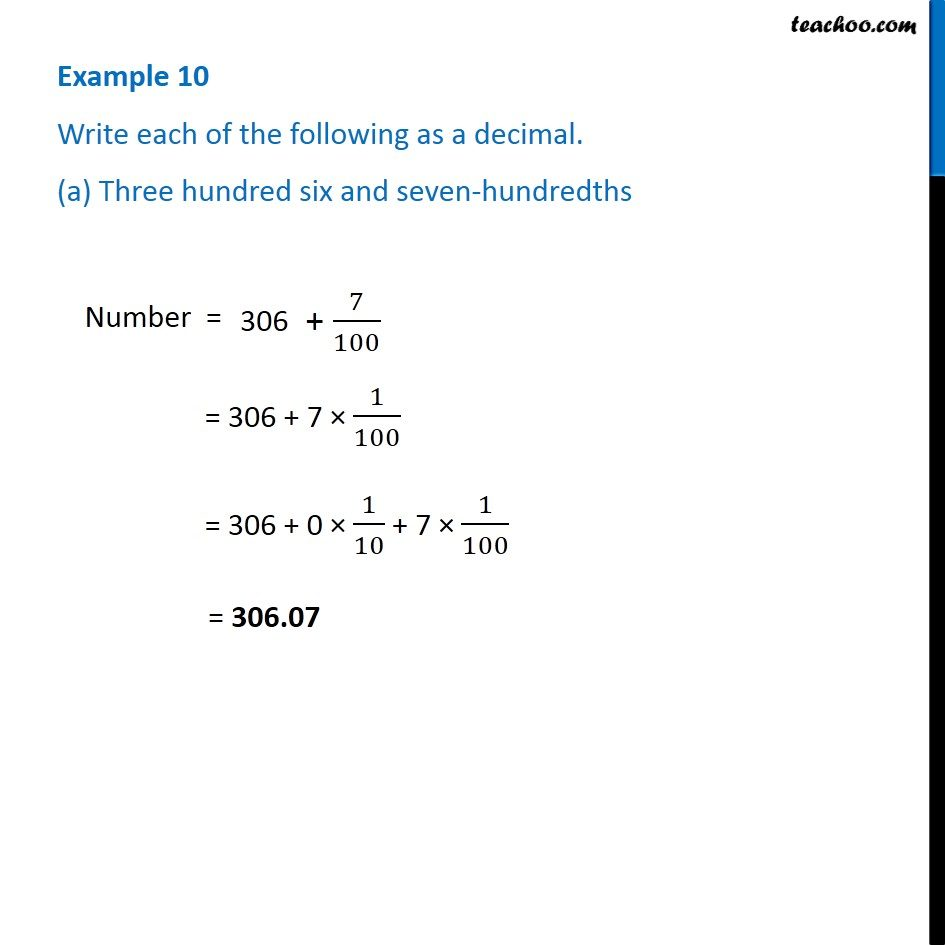1. Chapter 8 Class 6 Decimals
2. Concept wise
3. Words to decimals

Transcript

Example 10 Write each of the following as a decimal. (a) Three hundred six and seven-hundredths Number = = 306 + 7 × 1/100 = 306 + 0 × 1/10 + 7 × 1/100 = 306.07